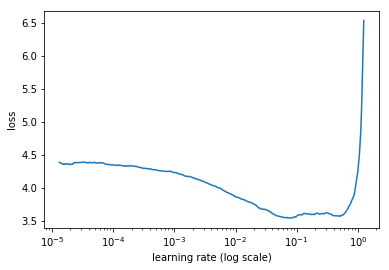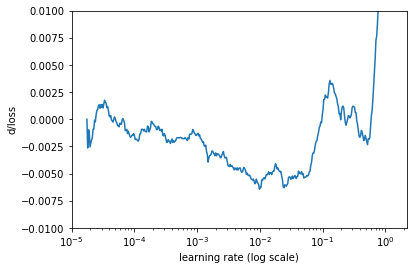Showing:

0

222

1yr ago

10

2

MIT

# keras_lr_finder

Plots the change of the loss function of a Keras model when the learning rate is exponentially increasing.

## Usage

Create and compile a Keras model, then execute this code:

``````# model is a Keras model
lr_finder = LRFinder(model)

# Train a model with batch size 512 for 5 epochs
# with learning rate growing exponentially from 0.0001 to 1
lr_finder.find(x_train, y_train, start_lr=0.0001, end_lr=1, batch_size=512, epochs=5)
``````
``````# Plot the loss, ignore 20 batches in the beginning and 5 in the end
lr_finder.plot_loss(n_skip_beginning=20, n_skip_end=5)
````````````# Plot rate of change of the loss
# Ignore 20 batches in the beginning and 5 in the end
# Smooth the curve using simple moving average of 20 batches
# Limit the range for y axis to (-0.02, 0.01)
lr_finder.plot_loss_change(sma=20, n_skip_beginning=20, n_skip_end=5, y_lim=(-0.01, 0.01))
``````## Contributions

Contributions are welcome. Please, file issues and submit pull requests on GitHub, or contact me directly.

This code is based on:

## Rate & Review

Great Documentation0
Easy to Use0
Performant0
Highly Customizable0
Bleeding Edge0
Responsive Maintainers0
Poor Documentation0
Hard to Use0
Slow0
Buggy0
Abandoned0
Unwelcoming Community0
100